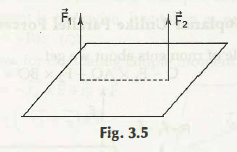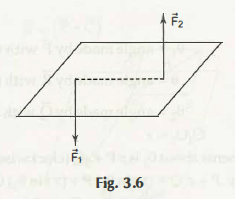Coplanar forces are the forces which are mainly implied on a single plane.

The notion mentioned above states that all forces are running equivalent to the plane and the points of application are available inside the plane. The force of coplanar can be of different types and they can be non-parallel or parallel, non-concurrent and concurrent, etc.

In this content it is important to mention that the force having concurrent type can be comprises of minimum 2 forces in which all the lines of the action merge together in action that too at a given point which is common for all.

It is equally important to add that a parallel force is having minimum of 2 different forces in which all the action lines will be parallel. Now, the most important thing which is the types of coplanar parallel forces.

Basically, there are 2 different types of coplanar parallel forces and they are discussed below.

1. Like Coplanar Parallel Forces

From the image given above you can see that force F1 and F2 moves in the same magnitude, now this is called like coplanar parallel forces.

(Refer Fig. 3.5)2. Unlike Coplanar Parallel Forces

A force is said to be unlike coplanar parallel force when there will be 2 different coplanar parallel forces and they act in reverse direction. You can check out the image given below.

(Ref. Fig. 3.6)Links of Previous Main Topic:-

Links of Next Mechanical Engineering Topics:-

### Submit Your Assignment### Customer Reviews

My Homework Help
Rated 5.0 out of 5 based on 510 customer reviews at